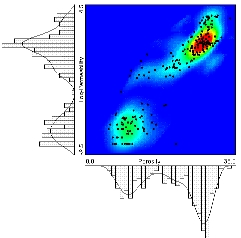GSLIB Help Page: BIVPLTDescription:
• The histsmth and scatsmth programs smooth univariate and bivariate distributions. The bivplt program documented here displays the results. The plot also shows the original data pairs and the original unsmoothed data histogram.Parameters:
• datafl: The data file with the paired data in a simplified Geo-EAS format.
• icolx, icoly, and icolwt: The columns for the x variable (horizontal axis), y variable (vertical axis), and weight (if less than or equal to zero then equal weighting is used; otherwise, the weight is used to calculate the summary statistics).
• ilogx and ilogy: set to 1 if log scaling has been used for the x or y axis respectively.
• tmin and tmax: all values strictly less than tmin and strictly greater than tmax are ignored.
• smoothx: the data file with the smoothed x distribution.
• icolvr and icolwt: the column location for the variable and the weight (probability).
• smoothy: the data file with the smoothed y distribution.
• icolvr and icolwt: the column location for the variable and the weight (probability).
• smoothxy: the data file with the smoothed bivariate distribution.
• icolx, icoly and icolwt: the column locations for the x and y variables and the weight (probability).
• outfl: file for PostScript output.
• imap and icolor: specify whether a pixel map of the bivariate probabilities will be created (imap1 will create one) and whether it will be color (icolor1) or gray scale ( icolor=0).
• pmin, pmax and pinc: minimum and maximum plotting limits for the pixel map.
• title: a 40-character title for the top of the plot.